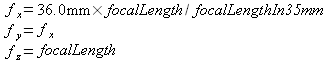Hauptmenü

Support > Manual

# Appendix A: Description of the projection model

## Projective collinearity equations

In the literature, there exists several formal description of the camera orientation. To clarify which notation is used in the export tables, we give here the formal definition of the camera orientation.

For the camera orientation, we use a 7 parameter model. A focal scaling parameter, the rotation of the camera and a translation vector. The focal scaling and rotation is expressed in terms of a quaternionand the translation is given by the vector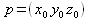.

The focal scaling is the squared norm of the quaternion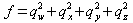and the rotation matrix is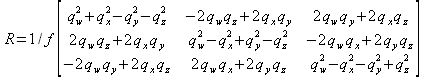The mapping of an object point located at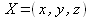to angular screen coordinatesof a pinhole camera is given by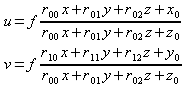.

The relation between image pixel (i, j) and angular coordinates (u, v) is given by.

The pixel coordinate (i_center, j_center) are the coordinates of the principle points of the image. The pixel numbering starts at the top left.

The focal resolution is expressed by the parameter fx, fy. The focal length is f_z.

Only very few camera vendors explicitly specify the exact focal resolution in the EXIF file format. Most vendors specify the focal resolution in terms of a 35mm equivalent.

In this case, we assume a square pixel size and the focal resolution is estimated as: